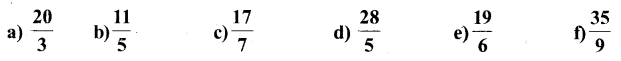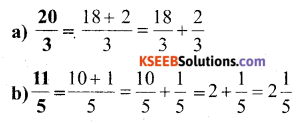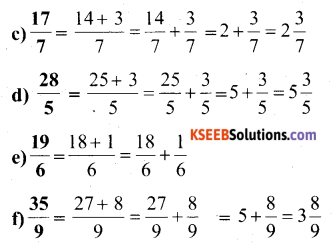KSEEB Solutions for Class 6 Maths Chapter 7 Fractions Ex 7.2

Students can Download Chapter 7 Fractions Ex 7.2 Questions and Answers, Notes Pdf, KSEEB Solutions for Class 6 Maths helps you to revise the complete Karnataka State Board Syllabus and score more marks in your examinations.

Karnataka State Syllabus Class 6 Maths Chapter 7 Fractions Ex 7.2

Question 1.
Draw number lines and locate the points on them:Solution:Question 2.
Express the following as mixed fraction:Solution:Question 3.
Express the following as improper fractions:Solution:error: Content is protected !!